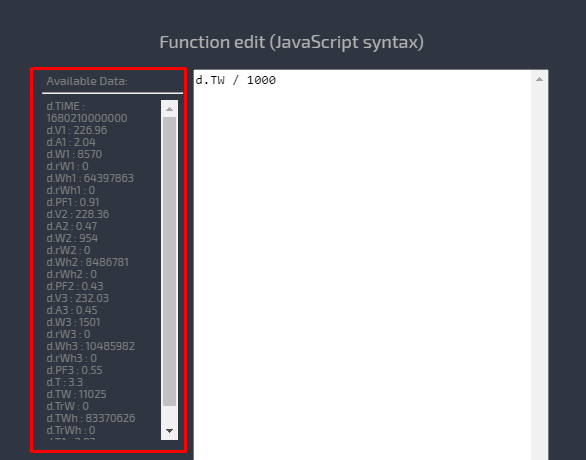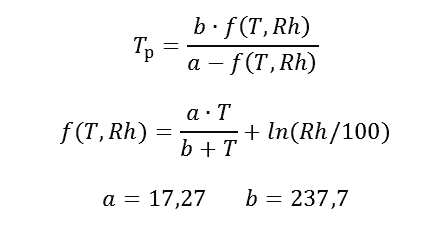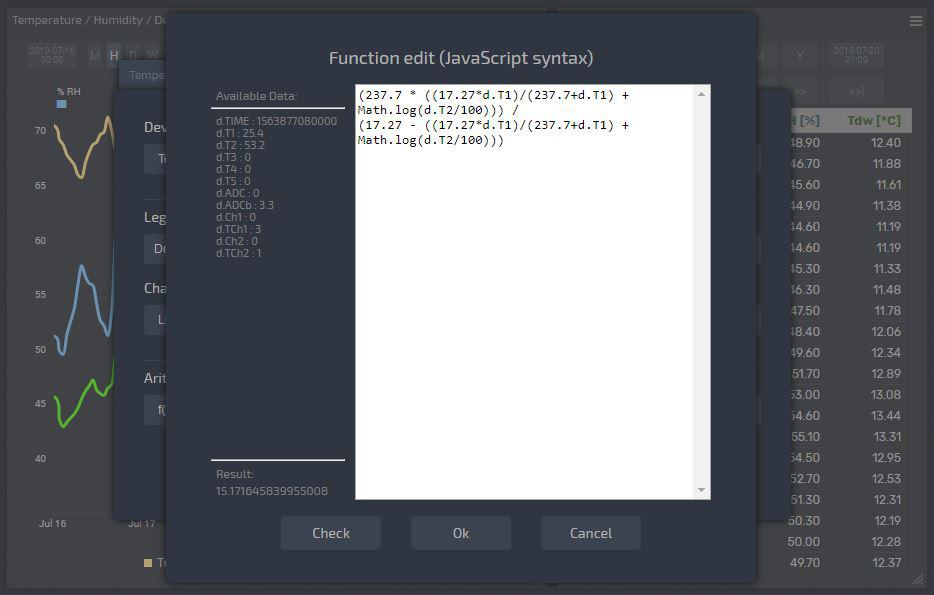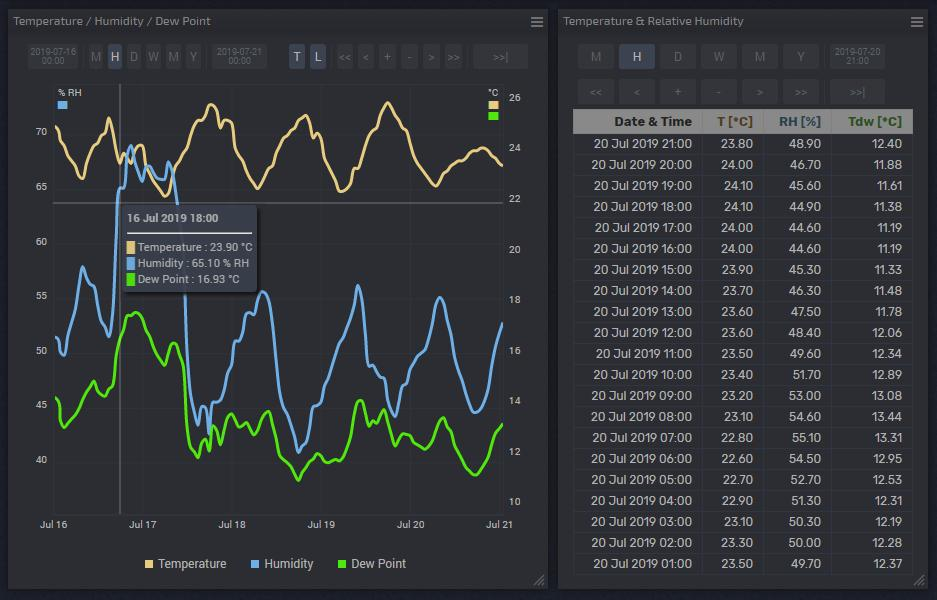# Complicated Functions in the widget settings

#### Complicated functions instead of simple Arithmetic operations

In the widget's settings, there is a section "Arithmetic operation". It seems easy for multiplied or divided by the coefficient and got the desired value, for example, divided Watts by 1000 and got Kilo-Watts. But among the arithmetic operations, there was a powerful tool f(x) with which you can describe any parameter based on the data that your smart meter monitors, for example, reactive energy in VAR, generated heat in Kilo-Watts or dew point in degrees Celsius. When calculating such indicators a simple arithmetic operation is not enough, usually, it is a formula and quite complicated.
This is where the f(x) tool comes to help in the "Arithmetic operation" settings, it is easier to use than it seems.

The main idea of ​​the tool f (x): you can write any complicated formula to get any new parameters using the parameters measured by the device and the JavaScript syntax.

Use the parameters measured by the device to construct formulas, in the format below:

• d.W1 - Power for 1st line;
• d.V2 - Voltage for 2nd line;
• d.A3 - Current for 3rd line;
• and so on.

The list of available parameters is presented on the left side in the formula editing window.

Important, the register of symbols matters!You also need to remember the rules of expression construction in mathematics and the order of calculation:

1. Actions written in brackets;
2. Multiplication and division;

For complex calculations, there is the Math library, which will provide you with trigonometric functions, power, roots, and logarithms. Below is a list of the Math object, which includes mathematical constants and functions in its properties and methods.

• Math.floor(a) rounds to the next smallest integer;
• Math.abs(a) returns the absolute value of a;
• Math.pow(a, b) computes a to the power of b;
• Math.sqrt(a) computes the square root of a;
• Math.log(a) computes the natural logarithm of a;
• Math.sin(a) calculates the sine of angle a;
• Math.cos(a) computes the cosine of a;
• Math.tan(a) calculates the tangent of angle a;
• Math.asin(a) calculates arcsine of a;
• Math.acos(a) arccosine of a;
• Math.atan(a) arctangent of a;

There is also a list of useful constants.:

• Math.PI (PI): 3.141592653589793
• Math.SQRT2 (the square root of two): 1.4142135623730951
• Math.SQRT1_2 (half of the square root of two): 0.7071067811865476
• Math.E (e or Euler number): 2.718281828459045
• Math.LN2 (natural logarithm of 2): 0.6931471805599453
• Math.LN10 (natural logarithm of 10): 2.302585092994046
• Math.LOG2E (the binary logarithm of e): 1.4426950408889634
• Math.LOG10E (the decimal logarithm of e): 0.4342944819032518

To check the correctness of the calculations, use the "Check" button, which will perform the calculation with the listed parameter values.

For example, will build a “Dew Point” graph based on Temperature and Humidity, which can be easily measured using the smart-MAIC D105.

The formula for finding the Dew Point is not so simple:

####But in the widget settings, it looks not so scary.

`(237.7 * ((17.27*d.T1)/(237.7 + d.T1) + Math.log(d.T2/100))) / (17.27 - ((17.27*d.T1)/(237.7+d.T1) + Math.log(d.T2/100)))`

Where: d.T1 is the measured Temperature and d.T2 is the measured Relative Humidity.